# NCERT Solutions for Class 9 Maths Chapter 11 Constructions| PDF Download

Chapter 11 Constructions Class 9 Maths NCERT Solutions will be help you in knowing variety of concepts and developing problem solving skills. You can download PDF of Chapter 11 Constructions NCERT Solutions for Class 9 Maths from this page through which one can study at their ease. These Chapter 11 NCERT Solutions will help you in completing homework in no time and make aware of the difficulty of questions.

Class 9 Maths NCERT Solutions will be useful in building your own answers and getting good marks in the examination. These solutions are updated according to the latest NCERT Class 9 Maths textbook. After understanding the important concepts of the chapter, a student can minimise their errors.Page No: 191

Exercise 11.1

1. Construct an angle of 90° at the initial point of a given ray and justify the construction.Steps of construction:

Step 1: A ray YZ is drawn.
Step 2: With Y as a centre and any radius, an arc ABC is drawn cutting YZ at C.
Step 3: With C as a centre and the same radius, mark a point B on the arc ABC.
Step 4: With B as a centre and the same radius, mark a point A on the arc ABC.
Step 5: With A and B as centre, draw two arcs intersecting each other with the same radius at X.
Step 6: X and Y are joined and a ray XY making an angle 90° with YZ is formed.

Justification for construction:
We constructed ∠BYZ = 60° and also ∠AYB = 60°.
Thus, ∠AYZ = 120°.
Also, bisector of ∠AYB is constructed such that:
∠AYB = ∠XYA + ∠XYB
⇒ ∠XYB = 1/2∠AYB
⇒ ∠XYB = 1/2×60°
⇒ ∠XYB = 30°
Now,
∠XYZ = ∠BYZ + ∠XYB = 60° + 30° = 90°

2. Construct an angle of 45° at the initial point of a given ray and justify the construction.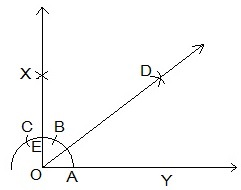Steps of construction:

Step 1: A ray OY is drawn.
Step 2: With O as a centre and any radius, an arc ABC is drawn cutting OY at A.
Step 3: With A as a centre and the same radius, mark a point B on the arc ABC.
Step 4: With B as a centre and the same radius, mark a point C on the arc ABC.
Step 5: With A and B as centre, draw two arcs intersecting each other with the same radius at X.
Step 6: X and Y are joined and a ray making an angle 90° with YZ is formed.
Step 7: With A and E as centres, two arcs are marked intersecting each other at D and the bisector of ∠XOY is drawn.

Justification for construction:
By construction,
∠XOY = 90°
We constructed the bisector of ∠XOY as DOY.
Thus,
∠DOY = 1/2 ∠XOY
∠DOY = 1/2×90° = 45°

3. Construct the angles of the following measurements:
(i) 30°        (ii) 22.5°        (iii) 15°

(i) 30°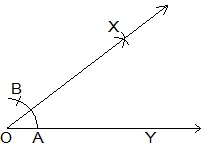Steps of constructions:
Step 1: A ray OY is drawn.
Step 2: With O as a centre and any radius, an arc AB is drawn cutting OY at A.
Step 3: With A and B as centres, two arcs are marked intersecting each other at X and the bisector of is drawn.
Thus, ∠XOY is the required angle making 30° with OY.

(ii) 22.5°Steps of constructions:
Step 1: An angle ∠XOY = 90° is drawn.
Step 2: Bisector of ∠XOY is drawn such that ∠BOY = 45° is constructed.
Step 3: Again, ∠BOY is bisected such that ∠AOY is formed.
Thus, ∠AOY is the required angle making 22.5° with OY.

(iii) 15°Steps of constructions:
Step 1: An angle ∠AOY = 60° is drawn.
Step 2: Bisector of ∠AOY is drawn such that ∠BOY = 30° is constructed.
Step 3: With C and D as centres, two arcs are marked intersecting each other at X and the bisector of ∠BOY is drawn.
Thus, ∠XOY is the required angle making 15° with OY.

4. Construct the following angles and verify by measuring them by a protractor:
(i) 75°         (ii) 105°        (iii) 135°

(i) 75°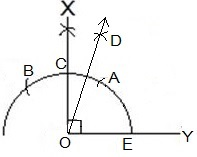Steps of constructions:
Step 1: A ray OY is drawn.
Step 2: An arc BAE is drawn with O as a centre.
Step 3: With E as a centre, two arcs are A and C are made on the arc BAE.
Step 4: With A and B as centres, arcs are made to intersect at X and ∠XOY = 90° is made.
Step 5: With A and C as centres, arcs are made to intersect at D
Step 6: OD is joined and and ∠DOY = 75° is constructed.
Thus, ∠DOY is the required angle making 75° with OY.

(ii) 105°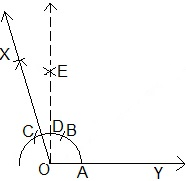Steps of constructions:
Step 1: A ray OY is drawn.
Step 2: An arc ABC is drawn with O as a centre.
Step 3: With A as a centre, two arcs are B and C are made on the arc ABC.
Step 4: With B and C as centres, arcs are made to intersect at E and ∠EOY = 90° is made.
Step 5: With B and C as centres, arcs are made to intersect at X
Step 6: OX is joined and and ∠XOY = 105° is constructed.
Thus, ∠XOY is the required angle making 105° with OY.

(iii) 135°Steps of constructions:Step 1: A ray DY is drawn.
Step 2: An arc ACD is drawn with O as a centre.
Step 3: With A as a centre, two arcs are B and C are made on the arc ACD.
Step 4: With B and C as centres, arcs are made to intersect at E and ∠EOY = 90° is made.
Step 5: With F and D as centres, arcs are made to intersect at X or bisector of ∠EOD is constructed.
Step 6: OX is joined and and ∠XOY = 135° is constructed.
Thus, ∠XOY is the required angle making 135° with DY.

5. Construct an equilateral triangle, given its side and justify the construction.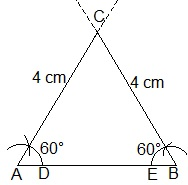Steps of constructions:
Step 1: A line segment AB=4 cm is drawn.
Step 2: With A and B as centres, two arcs are made.
Step 4: With D and E as centres, arcs are made to cut the previous arc respectively and forming angle of 60° each.
Step 5: Lines from A and B are extended to meet each other at C.
Thus, ABC is the required triangle formed.

Justification:
By construction,
AB = 4 cm, ∠A = 60° and ∠B = 60°
We know that,
∠A + ∠B + ∠C = 180° (Sum of the angles of a triangle)
⇒ 60° + 60° + ∠C = 180°
⇒ 120° + ∠C = 180°
⇒ ∠C = 60°
BC = CA = 4 cm (Sides opposite to equal angles are equal)
AB = BC = CA = 4 cm
∠A = ∠B = ∠C = 60°

Page No: 195

Exercise 11.2

1. Construct a triangle ABC in which BC = 7cm, ∠B = 75° and AB + AC = 13 cm.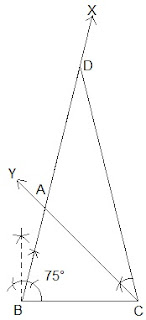Steps of Construction:
Step 1: A line segment BC of 7 cm is drawn.
Step 2: At point B, an angle ∠XBC is constructed such that it is equal to 75°.
Step 3: A line segment BD = 13 cm is cut on BX (which is equal to AB+AC).
Step 3: DC is joined and ∠DCY = ∠BDC is made.
Step 4: Let CY intersect BX at A.
Thus, ΔABC is the required triangle.

2. Construct a triangle ABC in which BC = 8cm, ∠B = 45° and AB - AC = 3.5 cm.Steps of Construction:
Step 1: A line segment BC = 8 cm is drawn and at point B, make an angle of 45° i.e. ∠XBC.
Step 2: Cut the line segment BD = 3.5 cm (equal to AB - AC) on ray BX.
Step 3: Join DC and draw the perpendicular bisector PQ of DC.
Step 4: Let it intersect BX at point A. Join AC.
Thus, ΔABC is the required triangle.

3. Construct a triangle PQR in which QR = 6cm, ∠Q = 60° and PR – PQ = 2cm.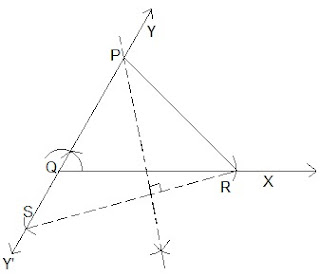Steps of Construction:
Step 1: A ray QX is drawn and cut off a line segment QR = 6 cm from it.
Step 2:. A ray QY is constructed making an angle of 60º with QR and YQ is produced to form a line YQY'
Step 3: Cut off  a line segment QS = 2cm  from QY'. RS is joined.
Step 5: Draw perpendicular bisector  of RS intersecting QY at a point  P. PR is joined.
Thus, ΔPQR is the required triangle.

4. Construct a triangle XYZ in which ∠Y = 30°, ∠Z = 90° and XY + YZ + ZX = 11 cm.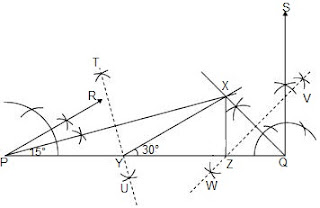Steps of Construction:
Step 1: A line segment PQ = 11 cm is drawn. (XY + YZ + ZX = 11 cm)
Step 2: An angle, ∠RPQ = 30° is constructed at point A and an angle ∠SQP = 90° at point B.
Step 3: ∠RPQ and ∠SQP are bisected . The bisectors of these angles intersect each other at point X.
Step 4: Perpendicular bisectors TU of PX and WV of QX are constructed.
Step V: Let TU intersect PQ at Y and WV intersect PQ at Z. XY and XZ are joined.
Thus, ΔXYZ is the required triangle.

5. Construct a right triangle whose base is 12cm and sum of its hypotenuse and other side is 18 cm.Steps of Construction:
Step 1: A ray BX is drawn and a cut off a line segment BC = 12 cm is made on it.
Step 2: ∠XBY = 90° is constructed.
Step 3: Cut off a line segment BD = 18 cm is made on BY. CD is joined.
Step 4: Perpendicular bisector of CD is constructed intersecting BD at A. AC is joined.
Thus, ΔABC is the required triangle.

## NCERT Solutions for Class 9 Maths Chapter 11 Constructions

NCERT Solutions of Chapter 11 Constructions provided here are detailed and accurate that will be give in depth study of concepts. Through this chapter you will learn some basic constructions and then after construct certain kinds of triangles.

• Basic Constructions: We will learn:
1. To construct the bisector of a given angle.
2. To construct the perpendicular bisector of a given line segment.
3. To construct an angle of 60° at the initial point of a given ray.

• Some Constructions of Triangles: We will learn:
1. To construct a triangle, given its base, a base angle and sum of other two sides.
2. To construct a triangle given its base, a base angle and the difference of the other two sides.
3. To construct a triangle, given its perimeter and its two base angles.

There are only two exercises in the whole chapter which will let you learn variety of constructions with given measures. You can always find exercisewise NCERT Solutions for Chapter 11 just by clicking on the links given below.

While preparing these NCERT Solutions for Class 9 Maths, Studyrankers experts have taken every care and provided a detailed step by step solutions of every question which will help you in learning the concepts embedded in the question.

### NCERT Solutions for Class 9 Maths Chapters:

 Chapter 1 Number Systems Chapter 2 Polynomials Chapter 3 Coordinate Geometry Chapter 4 Linear Equations in Two Variables Chapter 5 Introduction to Euclid’s Geometry Chapter 6 Lines and Angles Chapter 7 Triangles Chapter 8 Quadrilaterals Chapter 9 Areas of Parallelograms and Triangles Chapter 10 Circles Chapter 12 Heron's Formula Chapter 13 Surface Areas And Volumes Chapter 14 Statistics Chapter 15 Probability

FAQ on Chapter 11 Constructions

#### Why we should solve NCERT Solutions for Chapter 11 Constructions Class 9?

These NCERT Solutions will make you equipped with variety of concepts which can be helpful in solving questions in the examinations. Also, our subject matter experts have prepared these Chapter 11 NCERT Solutions as per the latest marking scheme which let you score more marks in tests.

#### What are the instruments required for drawing geometrical figures?

A protractor, a pair of compasses, a pair of set squares, a pair of dividers, a graduated scale are required  instruments for drawing geometrical figures.

#### How many exercises in Chapter 11 Constructions?

Chapter 11 Constructions consists of two exercises. In the first one, you will learn to draw basic constructions such as constructing a bisector while in the second one you will have to draw triangles with given measures.

#### How can I understand the topics given in Chapter 11 Class 9 Maths?

It is very necessary to understand the topics present in Chapter 11 Class 9 Maths and NCERT Solutions is one of the best way of doing. By practicing questions, you will get to know the concepts behind them and will make you ready for supplementary Maths Books of Class 9.